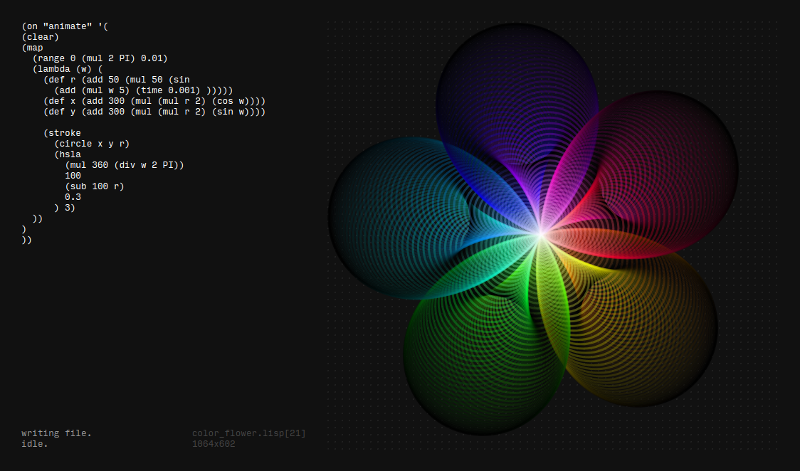## Ronin experiment 13 - color flower

I added two commands to the ronin library for creating colors (rgba ..) and (hsla ..), now it is a lot easier to animate colors or choose them programmaticallythis is the code I used to generate the flower

``````(on "animate" '(
(clear)
(map
(range 0 (mul 2 PI) 0.01)
(lambda (w) (
(def r (add 50 (mul 50 (sin
(add (mul w 5) (time 0.001) )))))
(def x (add 300 (mul (mul r 2) (cos w))))
(def y (add 300 (mul (mul r 2) (sin w))))

(stroke
(circle x y r)
(hsla
(mul 360 (div w 2 PI))
100
(sub 100 r)
0.3
) 3)
))
)
))
``````